kabeor 7月 25, 2019

# 逆向分析加解密之TwoFish算法

## 算法流程

http://www.schneier.com/twofish-analysis-shiho.pdf

### 流程图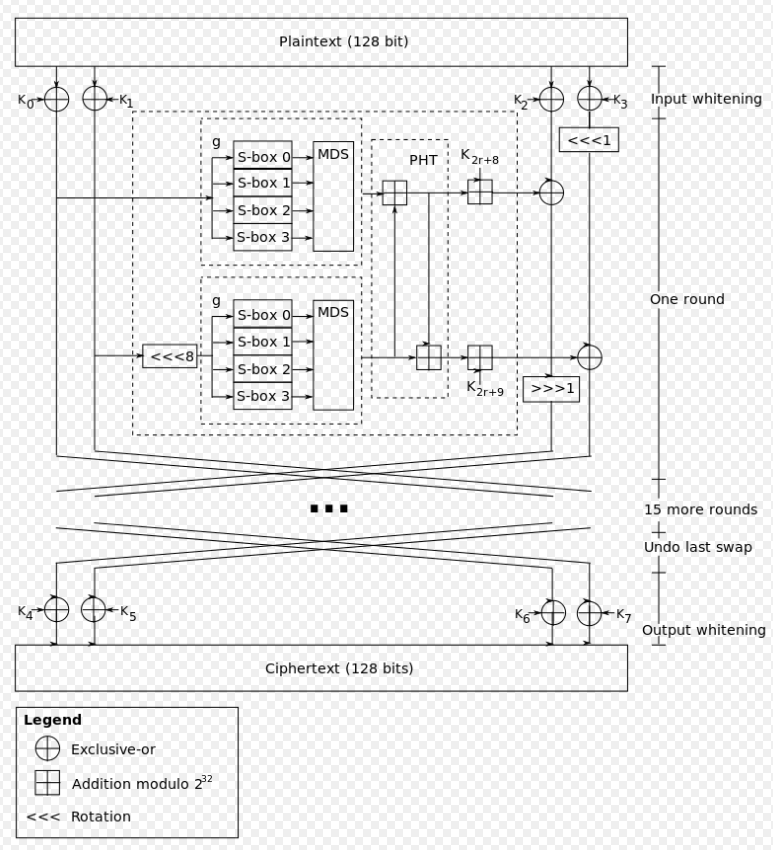TwoFish的意思应该就是这样交叉运算的形状吧

### 算法分析

TwoFish加密需要明文(plain)和密钥(key)

1. Input whitening
2. 16次循环
3. Output whitening

#### Input whitening

1. 拓展密钥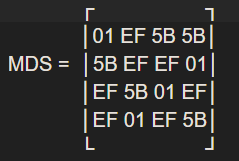`GF(2^8) ≡ GF(2)(x)/v(x)，其中v(x) = x^8 + x^6 + x^5 + x^3 + 1`

`````` y(k,j) = x(j)                     j = 0, ... ,3

y(3,0) = q1[y(4,0)] xor l(3,0)
y(3,1) = q0[y(4,1)] xor l(3,1)
y(3,2) = q0[y(4,2)] xor l(3,2)
y(3,3) = q1[y(4,3)] xor l(3,3)

y(2,0) = q1[y(3,0)] xor l(2,0)
y(2,1) = q1[y(3,1)] xor l(2,1)
y(2,2) = q0[y(3,2)] xor l(2,2)
y(2,3) = q0[y(3,3)] xor l(3,3)

y0 = q1[q0[q0[y(2,0)] xor l(1,0)] xor l(0,0)]
y1 = q0[q0[q1[y(2,1)] xor l(1,1)] xor l(0,1)]
y2 = q1[q1[q0[y(2,2)] xor l(1,2)] xor l(0,2)]
y3 = q0[q1[q1[y(2,3)] xor l(1,3)] xor l(0,3)]
``````

1. 输入白化

`P(i) = ∑p(4i+j)2^(8j)，其中i,j = 0, ... ,3`

#### 16次运算

``````(F(r,0), F(r,1)) = F(R(r,0), R(r,1), r)
R(r+1,0) = ROR(R(r,2) xor F(r,0), 1)
R(r+1,1) = ROL(R(r,3), 1) xor F(r,1)
R(r+1,2) = R(r,0)
R(r+1,3) = R(r,1)
``````

``````t0 = g(r0)
t1 = rol(r1, 8)
t1 = g(t1)
o = 2*r
F0 = (T0 +  T1 + K(2r+8)) mod 2^32
F1 = (T0 + 2T1 + K(2r+9)) mod 2^32
``````

``````x(i) = [X/2^(8i)] mod 2^8  其中i = 0, ... ,3
y(i) = s(i)(x(i))       其中i = 0, ... ,3
``````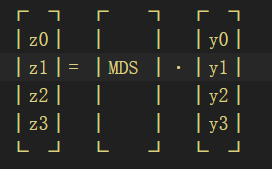``````Z = ∑z(i)2^(8i)，其中i = 0, ... ,3
``````

#### 输出白化

``````C(i) = R(16,(i+2) mod 4) xor K(i+4)，其中i = 0, ... ,3
``````

``````c(i) = [C(i/4) / 2^(8(i mod 4))] mod 2^8，其中i = 0, ... ,15
``````

## 逆向分析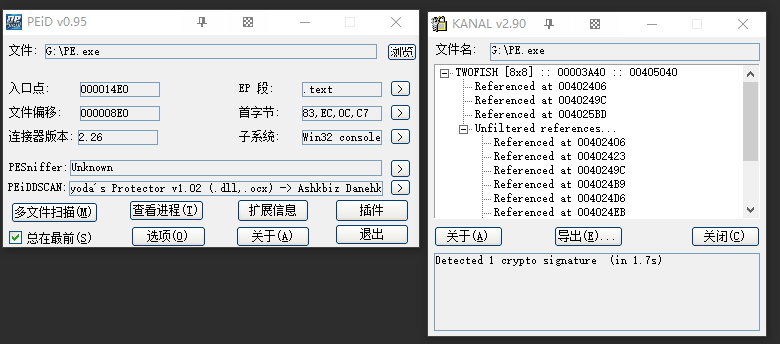IDA分析一下，进入主函数看到流程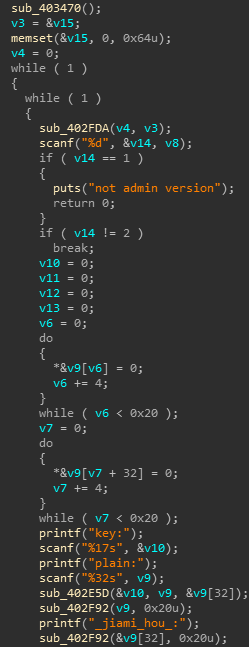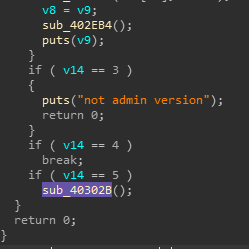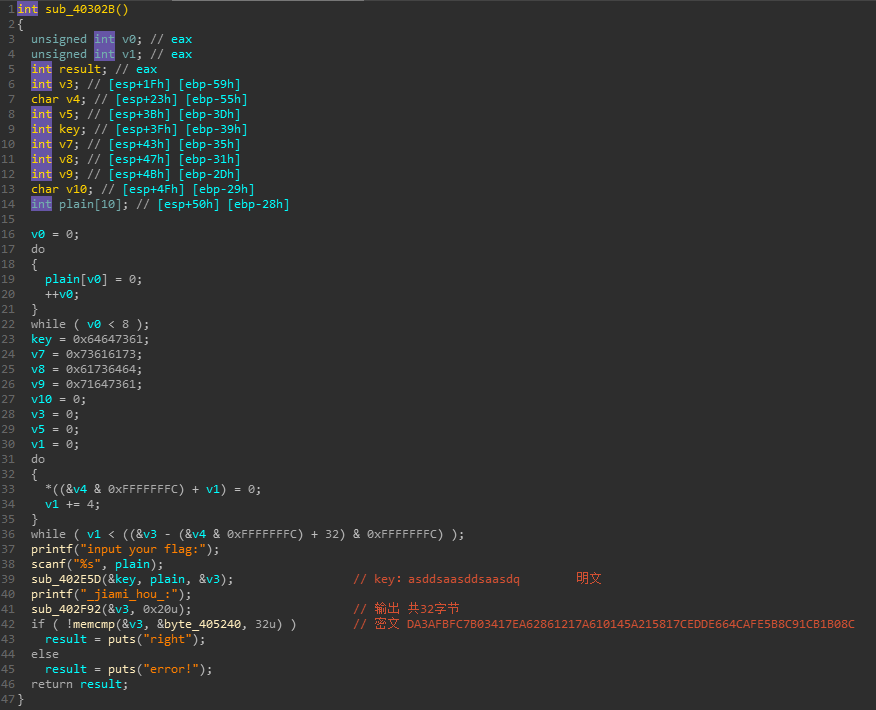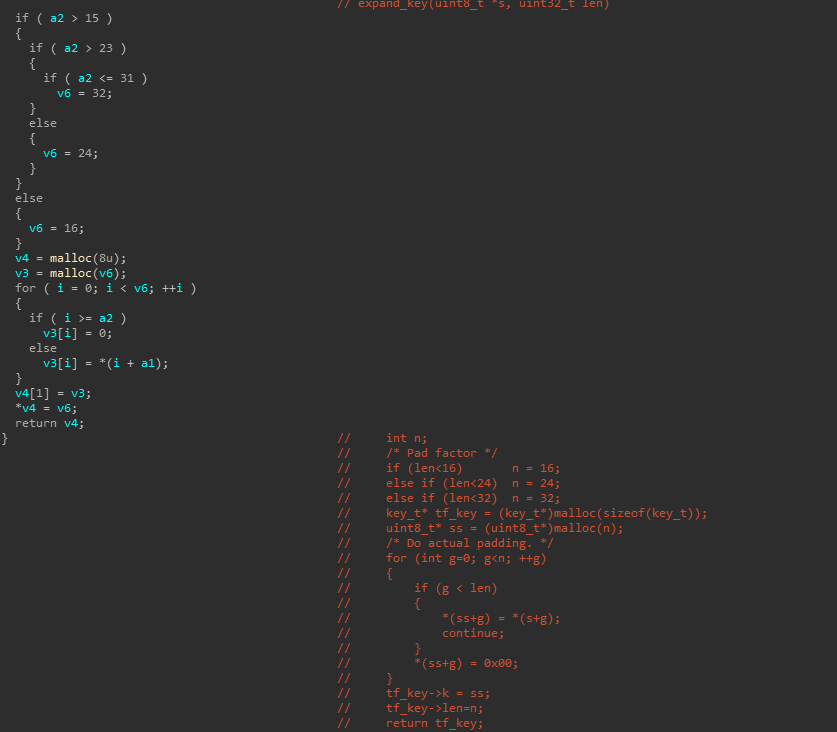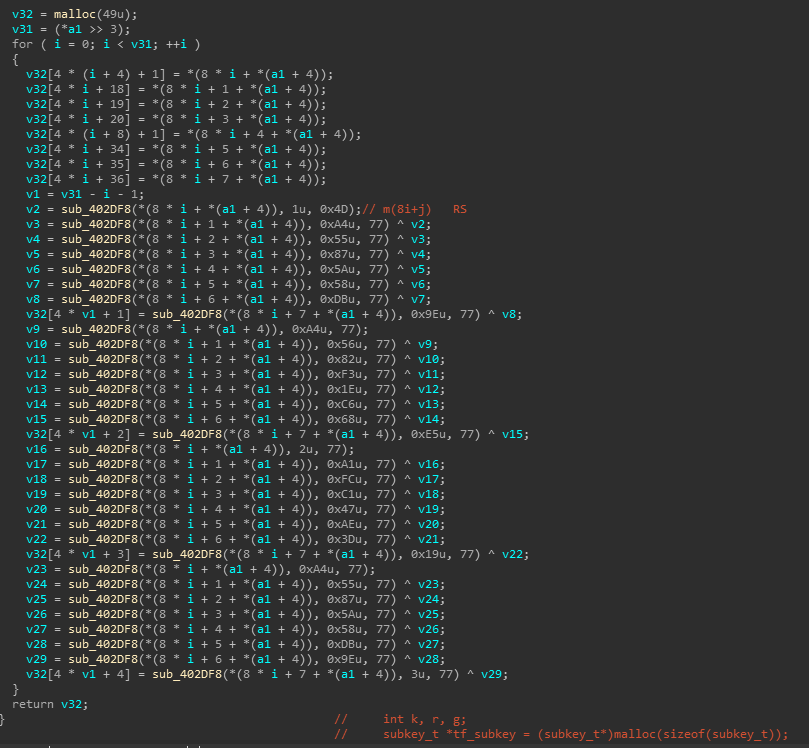c实现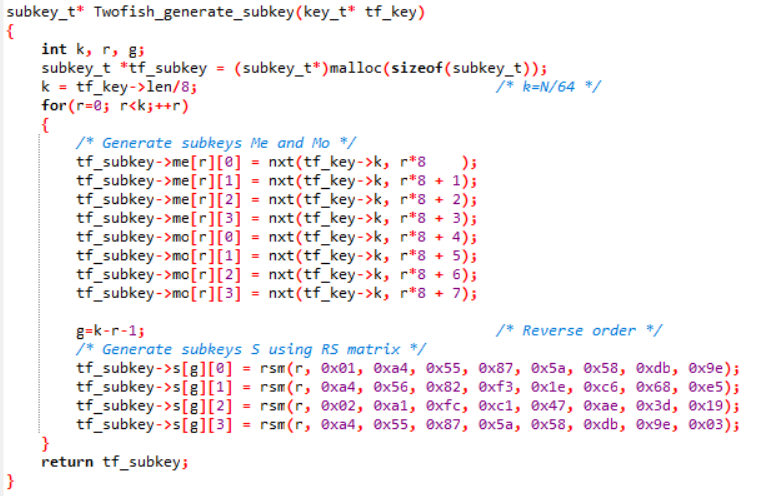rsm函数定义为

k生成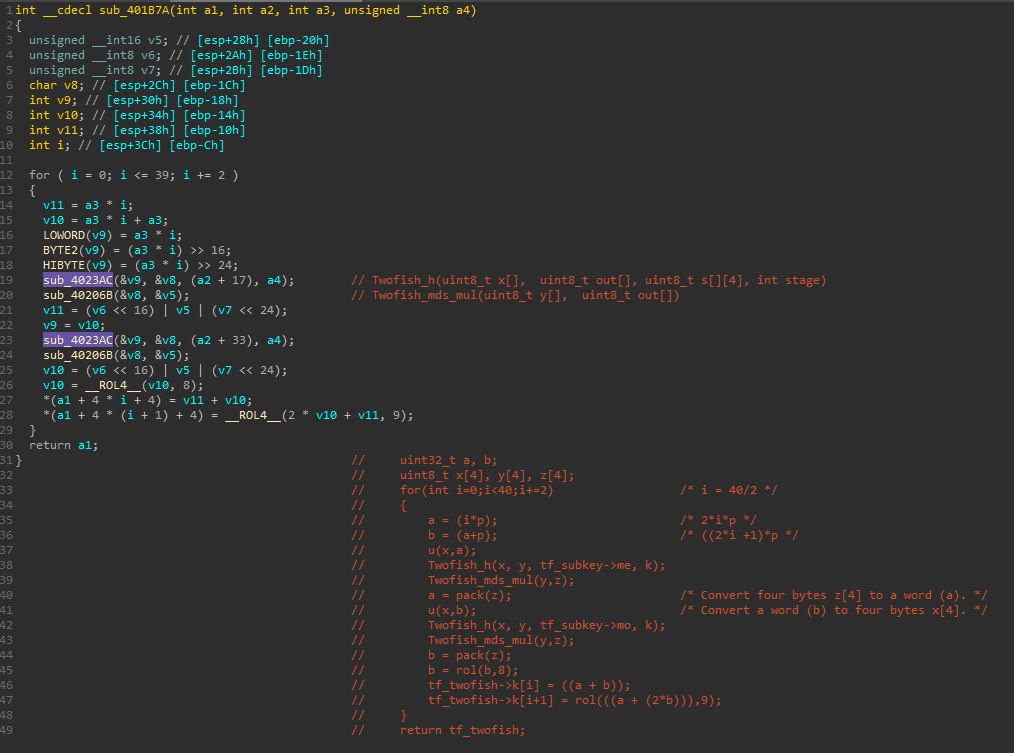h函数内部，可以看出，IDA将二维数组直接一维化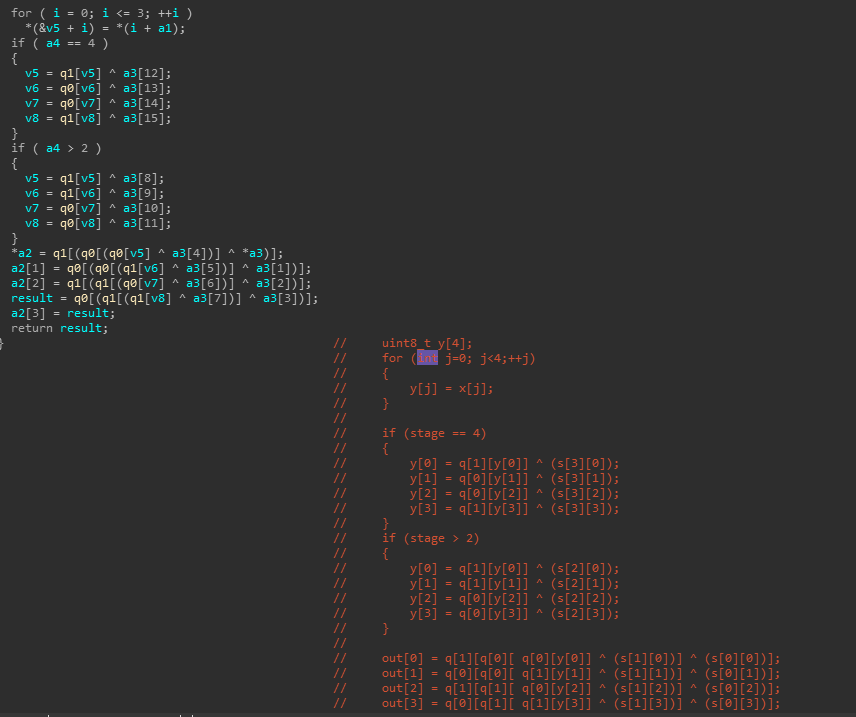q0,q1都是256大小的数组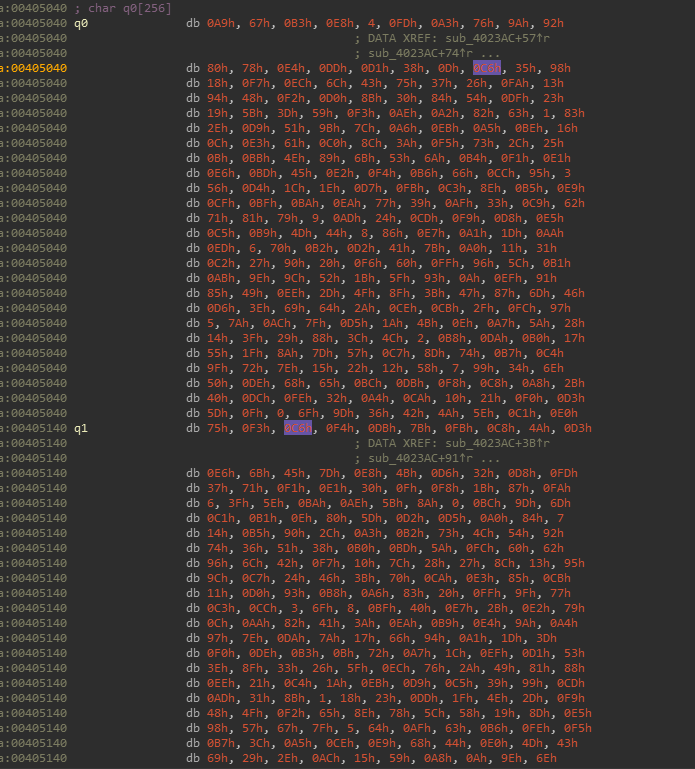MDS矩阵运算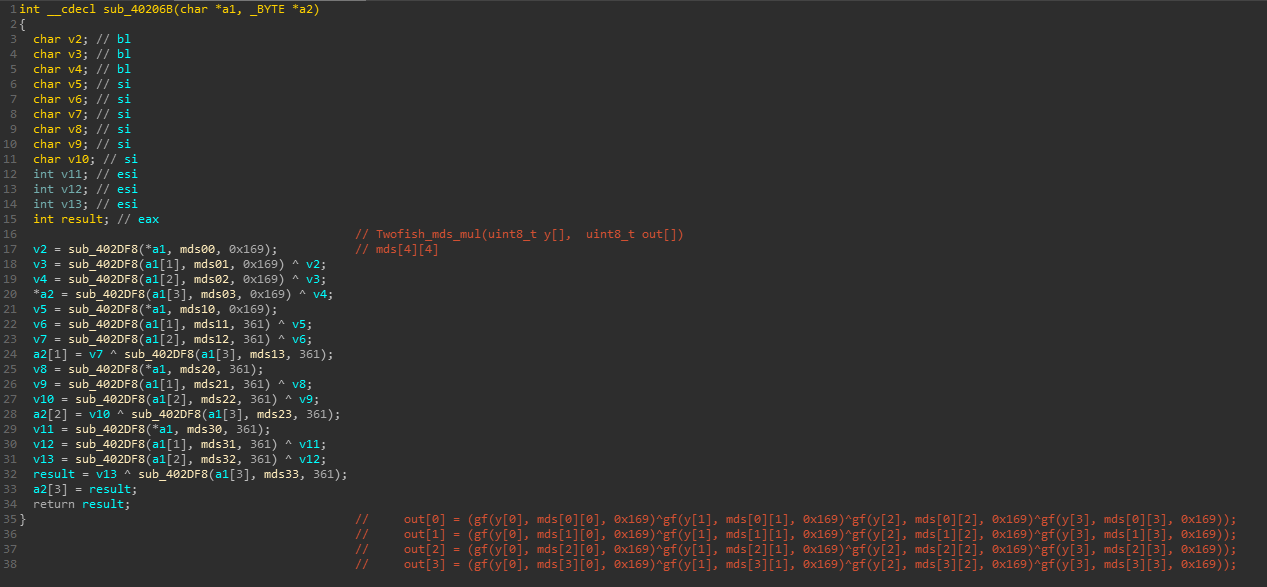S-box生成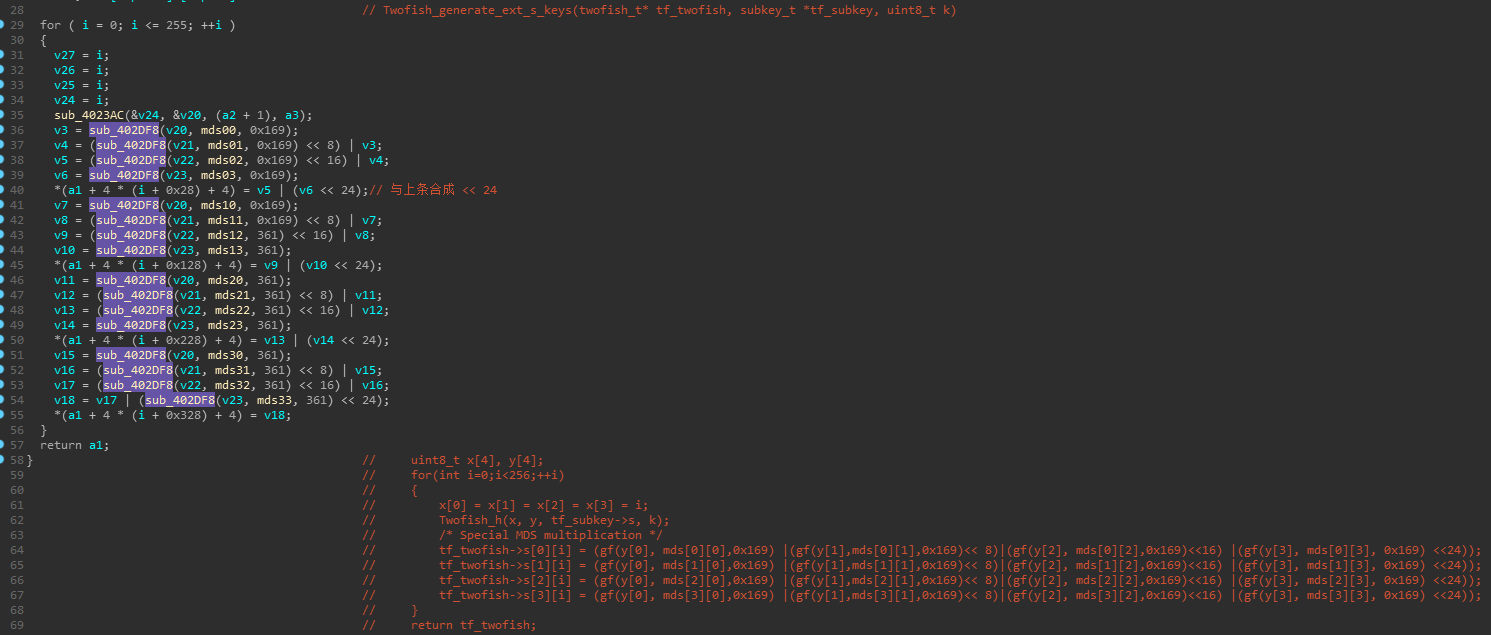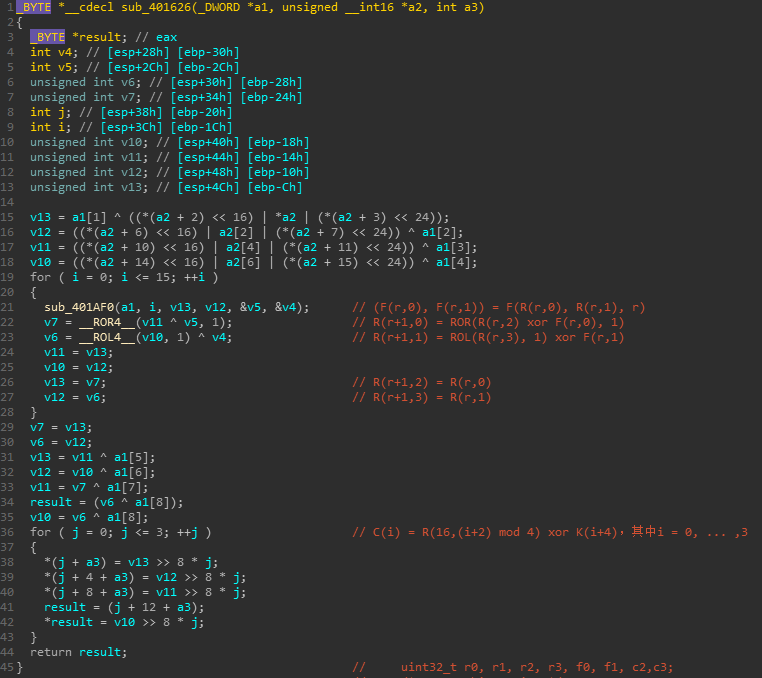c实现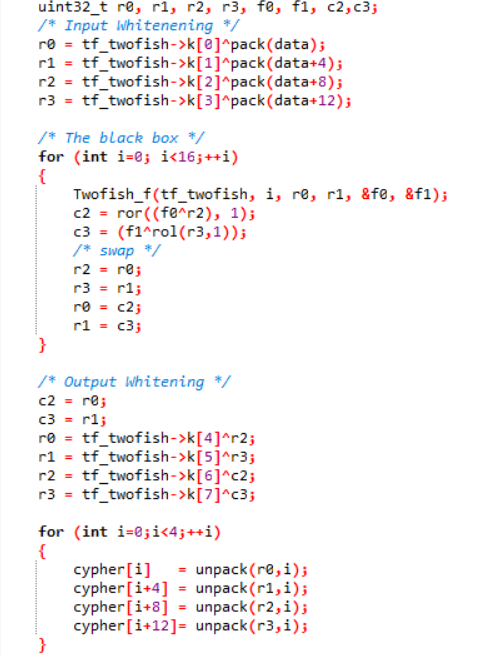f函数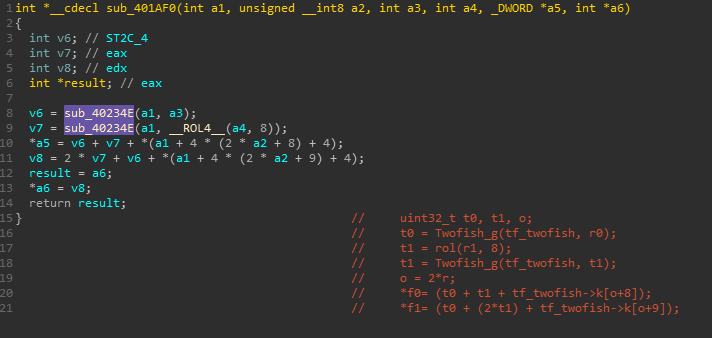twofish.h

tables.h

twofish.c

## CTF出题变化分析

TwoFish算法共有三处可发生变化以提高出题难度

1. rsm函数，0x14d可替换为其他数字
2. Twofish_generate_ext_s_keys函数中gf的参数0x166可替换
3. Twofish_mds_mul函数中gf的参数0x166可替换

This blog is under a CC BY-NC-SA 4.0 Unported License